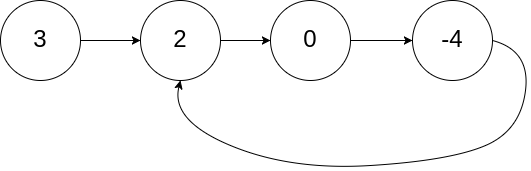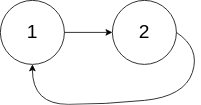## 描述

There is a cycle in a linked list if there is some node in the list that can be reached again by continuously following the next pointer.

Internally, pos is used to denote the index of the node that tail’s next pointer is connected to. Note that pos is not passed as a parameter.

Return true if there is a cycle in the linked list. Otherwise, return false.

## EX

Example 1:Input: head = [3,2,0,-4], pos = 1
Output: true
Explanation: There is a cycle in the linked list, where the tail connects to the 1st node (0-indexed).


Example 2:Input: head = [1,2], pos = 0
Output: true
Explanation: There is a cycle in the linked list, where the tail connects to the 0th node.


Example 3:

Input: head = , pos = -1
Output: false
Explanation: There is no cycle in the linked list.


## Constraints:

The number of the nodes in the list is in the range [0, 10^4].

-10^5 <= Node.val <= 10^5

pos is -1 or a valid index in the linked-list.

# 解题思路

## 第一感觉

1）pos 我们在入参中没有，但是题目又说到这个，令人困惑。

2）node 节点我们能得到的只有 value 值，但是值并不是唯一的。所以循环看值是否重复，这是不可行的。

## 快慢指针

fast 一次走2步，slow 一次走1步。

## java 解法

public boolean hasCycle(ListNode head) {
while (fast != null
&& fast.next != null) {
fast = fast.next.next;
slow = slow.next;
if(fast == slow) {
return true;
}
}
return false;
}


# 142. Linked List Cycle II

Given the head of a linked list, return the node where the cycle begins. If there is no cycle, return null.

There is a cycle in a linked list if there is some node in the list that can be reached again by continuously following the next pointer.

Internally, pos is used to denote the index of the node that tail’s next pointer is connected to (0-indexed). It is -1 if there is no cycle. Note that pos is not passed as a parameter.

Do not modify the linked list.

## EX

Example 1:Input: head = [3,2,0,-4], pos = 1
Output: tail connects to node index 1
Explanation: There is a cycle in the linked list, where tail connects to the second node.


Example 2:Input: head = [1,2], pos = 0
Output: tail connects to node index 0
Explanation: There is a cycle in the linked list, where tail connects to the first node.


Example 3:

Input: head = , pos = -1
Output: false
Explanation: There is no cycle in the linked list.


## Constraints:

The number of the nodes in the list is in the range [0, 10^4].

-10^5 <= Node.val <= 10^5

pos is -1 or a valid index in the linked-list.

# 第一感觉

1. 链表中是否存在环？

2. 如何找到环开始的节点？

java-o-1-space-solution-with-detailed-explanationq 点就是循环开始的节点，开始到 q 点的距离为 a

b 为 q 到 p 的距离

c 为 p 到 q 的距离。

## java 实现

public ListNode detectCycle(ListNode head) {
while (fast != null
&& fast.next != null) {
fast = fast.next.next;
slow = slow.next;
if(fast == slow) {
// 此时，slow2 从头开始，slow 从重合点开始。
while (slow != slow2) {
slow = slow.next;
slow2 = slow2.next;
}
// 两个慢指针重合时，slow2 就在第一个环的节点上
return slow2;
}
}
return null;
}